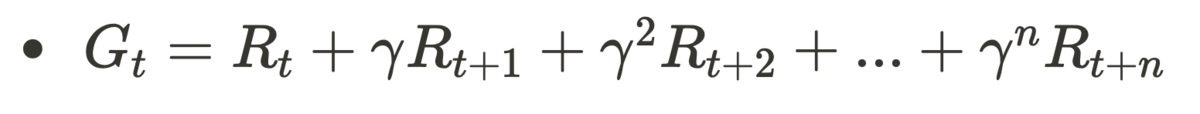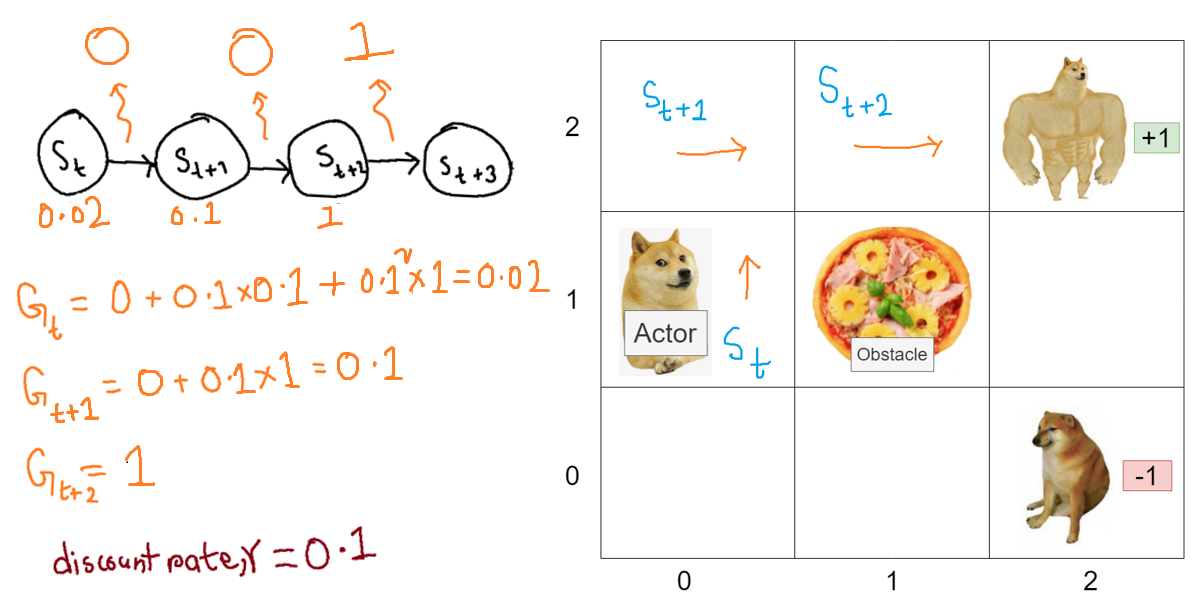# Markov Decision Processes

This is Shakil from Product Engineering Department. This time I wanted to talk a bit about Markov Decision Processes which forms the basis of Reinforcement Learning.

### What are MDPs (Markov Decision Processes)?

MDP provides a mathematical toolset to model Problems involving decision making in sequences where what the future state is going to be depends only on the actions taken from the present state.

### Why are MDPs interesting?

MDPs can be used to model and solve a wide range of problems, from Self-Driving Cars, to Recommendation Systems to Complex Video Games like DOTA 2.

Open AI 5 by DeepMind defeated human world Champions of DOTA 2 in 2019 by using MDPs as one of the tools to model and master the game.

### So how can we model a problem with MDPs?

in order to be modeled by a Markov Decision Process, a problem must must have the following properties.

• There must be Observable states
• There must be Actions an Agent/Actor can take to affect these Observed States
• The Probabilities of Transitioning to Future Observable States must only be dependent on the Previous State
• There must be a Reward Distribution to Indicate Goal States or Undesirable States

For example, in DOTA 2 a possible observable State, could be enemy having 9 remaining towers . An Action, could be attacking one of the towers and another State, would be the enemy having 8 towers remaining. Here, you can make a transition fromtotaking. Here, Transitioning todepends on being in stateto begin with. When transitioning fromtothe acting agent can get a small reward, for destroying an enemy tower as it has brought it closer to winning the game.

## Five Components of MDPs

• States: What you can observe
• Actions/Policy: Things you will be able to do in a state
• Transitions Function: Probabilities of transitioning from one state to another
•|,)
• Where t, represents timestep
• Rewards: Gold for reaching goals, Poison for falling into pit -• Discount Factor, γ: a value between 0 and 1
• γ ∈ [0, 1]

### States

A state is what an actor in an environment observes at a given timestep. The state should be defined such that it contains all the information that affects the transition to a future state from the current one. For example in the following Grid World environment, a state is one of the boxes in the grid. It can be represented as a coordinate like (0, 0).

### Policy/Action

An action Policy function gives us the probabilities the possible actions an Actor can take given a State,.

Formally Defined as:

-)- whereis the Policy Function

For example in the following figure, the Question is a State, and the Options A B C D are the possible actions that the Actor can take in this state.

### Rewards

In MDPs, positive rewards are used to push the Actor towards Good States, and negative rewards are used to pull the Actor away from bad states. For example in the following, the Actor gets a cookie for good behaviour and scolding for bad behaviour.

Formally Defined as:

-### State Transition Probabilities

Often problems we model with MDPs are non-deterministic i.e. taking the same action in the same state can take us to different future states as illustrated in the example below.

To model this non-determinism that is often seen in real life scenarios, MDP has a State Transition Probability Function which gives us the probability of transitioning to a State,, given our current stateand current Action.

Formally Defined as:

-### Discount Factor

γ ∈ [0, 1] is a discount factor representing the difference in importance between present and future rewards.

Discount Factor is a value is between 0 and 1. Discount factor basically acts as a weight which is used to reduce the importance of States and rewards received that are further away from the current State. More about this is discussed in the Aggregate Reward/Return section.

## Aggregate Reward / Return

The Aggregate Rewards or Return for a Stateis the sum of total rewards that an Actor receives from an Environment, starting from state, till the end of an Episode or until an certain timestep,.

Rewards that are further timesteps away from the State,for which the Return is calculated, are weighted lower by multiplying the discount factor (which as mentioned as before should be between 0 and 1). The Discount Factor is exponentiated the further timesteps away from statethe the reward was received. This is done so that the Actor gives more importance to States and rewards nearer to it.

Formally Aggregate Reward is defined as follows:- Whereis the Aggregate Return

In the Example Below, as the States get closer,, to the actual reward in green, their Aggregate Reward increases to indicate that a real large reward is nearby. While states that are further away,, get a smaller portion of the same reward due to the weighting the discount rate applies to rewards that are further timesteps away.## Conclusion

The purpose of modeling problems as MDP is to eventually find an optimal Action Policy for every State. An Optimal Action Policy is defined as a Policy that maximises the mean Cumulative reward that an actor receives over its lifetime.